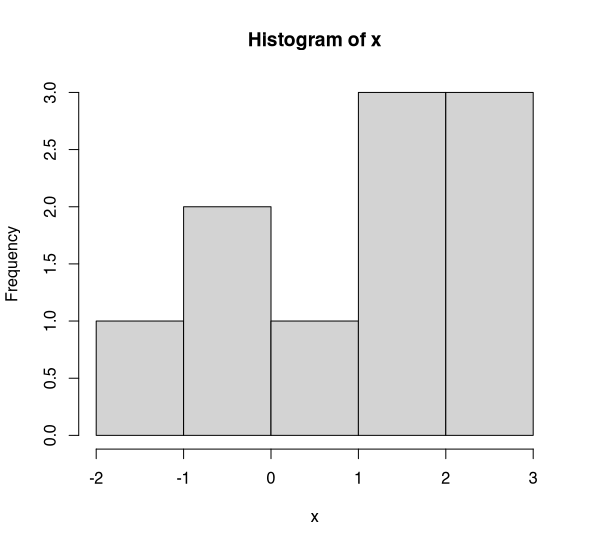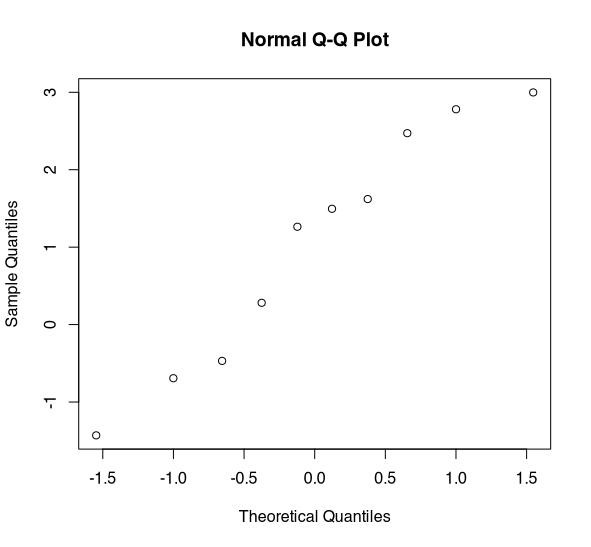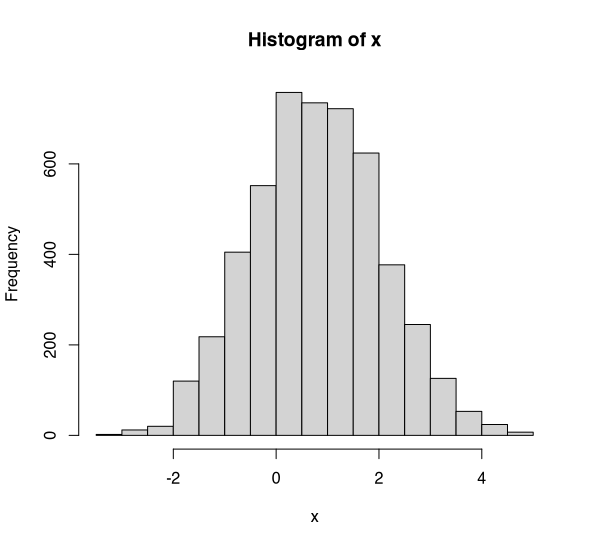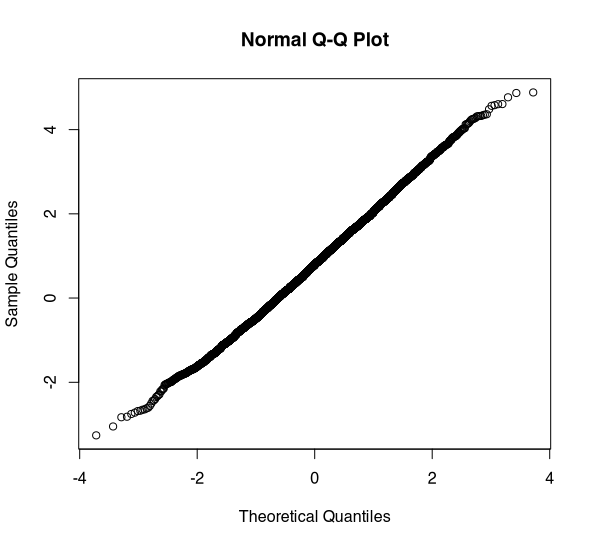1594896420

# Why is the Normal Distribution so Normal?

The Normal Distribution (or a Gaussian) shows up widely in statistics as a result of the Central Limit Theorem. Specifically, the Central Limit Theorem says that (in most common scenarios besides the stock market) anytime “a bunch of things are added up,” a normal distribution is going to result.

But why? Why that distribution? Why is it special? Why not some other distribution? Are there other statistical distributions where this happens?

Teaser: the answer is yes, there are other distributions that are special in the same way as the Normal distribution. The Normal distribution is still the most special because:

• It requires the least math
• It is the most common in real-world situations with the notable exception of the stock market

If you’re intrigued, read on! I’ll give an intuitive sketch of the Central Limit Theorem and a quick proof-sketch before diving into the Normal distribution’s oft-forgotten cousins.

# The Central Limit Theorem

Here is a quick official statement:

• Suppose you have n random variables X₁, X₂, … etc. representing a sample of size _n _from some population with population mean μ and finite variance σ². The population could follow any distribution at all.
• We are interested in their mean X, which itself a random variable. (It is random because each time we take a sample of size n, we get a different result).
• We already know that the mean X will have mean μ and variance σ²/n (this is true by the independence assumption and is a general property of random variables).
• The central limit theorem says that when _n _is large (usually 40+ is close enough in real life) the mean X follows a normal distribution, no matter what the distribution of underlying population is.

Formally,Formal statement of the Central Limit Theorem

Where Φ represents the normal distribution with mean and variance as given. (You may be used to seeing the equivalent standard deviation σ/√n instead). The “in distribution” is a technical bit about how the convergence works. We’ll ignore such technicalities from here on out.

## Why the Central Limit Theorem Shows Up

The Central Limit Theorem shows up in all sorts of places in real-world situations. For example, it’s a pretty reasonable assumption that your height can be expressed as the sum of a bunch of factors related to, among others:

1. How much milk you drank every day when you were 8 years old
2. How many X and/or Y chromosomes you have
3. Which variant of the GH1 gene you have
4. A whole bunch of other genes
5. Whether you slept in a Procrustean bed as a child

Take a whole bunch of factors, each of which makes a small difference in your final (adult) height, and, presto, you end up with a (roughly) normal distribution for human heights!

Note that I cheated slightly – the variables here aren’t i.i.d. But the independence assumption is a reasonable approximation, and there are stronger versions of the central limit theorem that relax the identical-distribution hypothesis. We did choose to leave out the cases of extreme genetic conditions that affect height however.

So in sum, any time something you measure is made up of a whole bunch of contributions from smaller parts being added up, you are likely to end up with a normal distribution.

## A Quick Proof

This proof is necessarily a sketch because, well, if you want a full proof with all of the analysis and probability theory involved, go read a textbook. The main point I want to get across is that there is a reason Euler’s constant _e _shows up.

First of all, we will need one high-powered mathematical tool. To every reasonable random variable X there is a_ characteristic function φ _which is, in essence, the Fourier Transform of the Probability-Density Function (PDF) of X.

#mathematics #central-limit-theorem #statistics #data-science #data analysis

## Buddha Community1624298520

## Introduction

In a series of weekly articles, I will be covering some important topics of statistics with a twist.

The goal is to use Python to help us get intuition on complex concepts, empirically test theoretical proofs, or build algorithms from scratch. In this series, you will find articles covering topics such as random variables, sampling distributions, confidence intervals, significance tests, and more.

At the end of each article, you can find exercises to test your knowledge. The solutions will be shared in the article of the following week.

Articles published so far:

As usual, the code is available on my GitHub.

#statistics #distribution #python #machine-learning #sampling distributions with python #sampling distributions1621248900

## What is Database Normalization in SQL Server – MS SQL Server – Zero to Hero Query Master

What is Database Normalization

Database normalization is the step by step process of organizing data to minimize data redundancy i.e. Data duplication which in turn ensures data consistency

• Normalization is a database design technique that reduces data redundancy and eliminates undesirable characteristics like Insertion, Update and Deletion Anomalies.
• Normalization rules divide larger tables into smaller tables and link them using relationships.
• The purpose of Normalization in SQL is to eliminate redundant (repetitive) data and ensure data is stored logically.
• The inventor of the relational model Edgar Codd proposed the theory of normalization of data with the introduction of the First Normal Form, and he continued to extend theory with Second and Third Normal Form. Later he joined Raymond F. Boyce to develop the theory of Boyce-Codd Normal Form.

#sql server #1nf #2nf #3nf #4nf #5nf #6nf #data #database in sql server #normalization #normalization forms #normalization in database #what is data1596896940

## Stop testing for normality

I see a lot of data scientists using tests such as the Shapiro-Wilk test and the Kolmogorov–Smirnov to test for normality. Stop doing this. Just stop. If you’re not yet convinced (and I don’t blame you!), let me show you why these are a waste of your time.

# Why do we care about normality?

We should care about normality. It’s an important assumption that underpins a wide variety of statistical procedures. We should always be sure of our assumptions and make efforts to check that they are correct. However, normality tests are not the way for us to do this.

However, in large samples (n > 30) which most of our work as data scientists is based upon the Central Limit Theorem usually applies and we need not worry about the normality of our data. But in cases where it does not apply let’s consider how we can check for normality in a range of different samples.

# Normality testing in small samples

First let us consider a small sample. Say n=10. Let’s look at the histogram for this data.Histogram of x (n=10). (Image by author)

Is this normally distributed? Doesn’t really look like it — does it? Hopefully you’re with me and accept that this isn’t normally distributed. Now let’s perform the Shapiro-Wilk test on this data.

Oh. p=0.53. No evidence to suggest that x is not normally distributed. Hmm. What do you conclude then. Well, of course, not being evidence that x is not normally distributed does not mean that x is normally distributed. What’s actually happening is that in small samples the tests are _underpowered _to detect deviations from normality.Normal Q-Q Plot of x (n=10). (Image by author)

The best way to assess normality is through the use of a quantile-quantile plot — Q-Q plot for short. If the data is normally distributed we would expect to see a straight line. This data shows some deviation from normality, the line is not very straight. There appears to be some issues in the tail. Admittedly, without more data it is hard to say.

With this data, I would have concerns about assuming normality as there appears to be some deviation in the Q-Q plot and in the histogram. But, if we had just relied on our normality test, we wouldn’t have picked this up. This is because the test is underpowered in small samples.

# Normality testing in large samples

Now let’s take a look at normality testing in a large sample (n=5000). Let’s take a look at a histogram.Histogram of x (n=5000). (Image by author)

I hope you’d all agree that this looks to be normally distributed. Okay, so what does the Shapiro-Wilk test say. Bazinga! p=0.001. There’s very strong evidence that x is not normally distributed. Oh dear. Well, let’s take a quick look at our Q-Q plot. Just to double check.Normal Q-Q plot for x (n=5000). (Image by author)

Wow. This looks to be normally distributed. In fact, there shouldn’t be any doubt that this is normally distributed. But, the Shapiro-Wilk test says it isn’t.

What’s going on here? Well the Shapiro-Wilk test (and other normality tests) are designed to test for theoretical normality (i.e. the perfect bell curve). In small samples these tests are underpowered to detect quite major deviations from normality which can be easily detected through graphical methods. In larger samples these tests will detect even extremely minor deviations from theoretical normality that are not of practical concern.

# Conclusion

Hopefully, I have shown you that normality tests are not of practical utility for data scientists. Don’t use them. Forget about them. At best, they are useless; at worst, they are misleading. If you want to assess the normality of some data, use Q-Q plots and histograms. They’ll give you a much clearer picture about the normality of your data.

#normal-distribution #statistics #tests-of-normality #mathematics #data-science1623263280

## Data Distribution in Apache Ignite

This blog is an abridged version of the talk that I gave at the Apache Ignite community meetup. You can download the slides that I presented at the meetup here. In the talk, I explain how data in Apache Ignite is distributed.

### Why Do You Need to Distribute Anything at all?

Inevitably, the evolution of a system that requires data storage and processing reaches a threshold. Either too much data is accumulated, so the data simply does not fit into the storage device, or the load increases so rapidly that a single server cannot manage the number of queries. Both scenarios happen frequently.

Usually, in such situations, two solutions come in handy—sharding the data storage or migrating to a distributed database. The solutions have features in common. The most frequently used feature uses a set of nodes to manage data. Throughout this post, I will refer to the set of nodes as “topology.”

The problem of data distribution among the nodes of the topology can be described in regard to the set of requirements that the distribution must comply with:

1. Algorithm. The algorithm allows the topology nodes and front-end applications to discover unambiguously on which node or nodes an object (or key) is located.
2. Distribution uniformity. The more uniform the data distribution is among the nodes, the more uniform the workloads on the nodes is. Here, I assume that the nodes have approximately equal resources.
3. Minimal disruption. If the topology is changed because of a node failure, the changes in distribution should affect only the data that is on the failed node. It should also be noted that, if a node is added to the topology, no data swap should occur among the nodes that are already present in the topology.

#tutorial #big data #distributed systems #apache ignite #distributed storage #data distribution #consistent hashing1623896372

## edistribucion

e-Distribución is an energy distribution company that covers most of South Spain area. If you live in this area, you probably are able to register into their website to get some information about your power demand, energy consumption, or even cycle billing (in terms of consumptions).

Although their application is great, this integration enables you to add a sensor to Home Assistant and getting updated automatically. However, it has some limitations yet, and no front-end support is being provided at the moment.

### How to install

1. Install HACS
2. Add this repo (https://github.com/uvejota/edistribucion) to the custom repositories in HACS
3. Install the integration. Please consider that alpha/beta versions are untested, and they might cause bans due to excesive polling.
4. Add this basic configuration at Home Assistant configuration files (e.g., `configuration.yml`)
``````sensor:
- platform: edistribucion
username: !secret eds_user ## this key may exist in secrets.yaml!
At this point, you got an unique default sensor for the integration, namely `sensor.edistribucion`, linked to those credentials in the e-Distribución platform. This default sensor assumes the first CUPS that appears in the fetched list of CUPS, which frequently is the most recent contract, so this configuration may be valid for most users. If you need a more detailed configuration, please check the section below “What about customisation?”.